# Question 21 of 33 What is AGº for a redox reaction where 6 moles of electrons...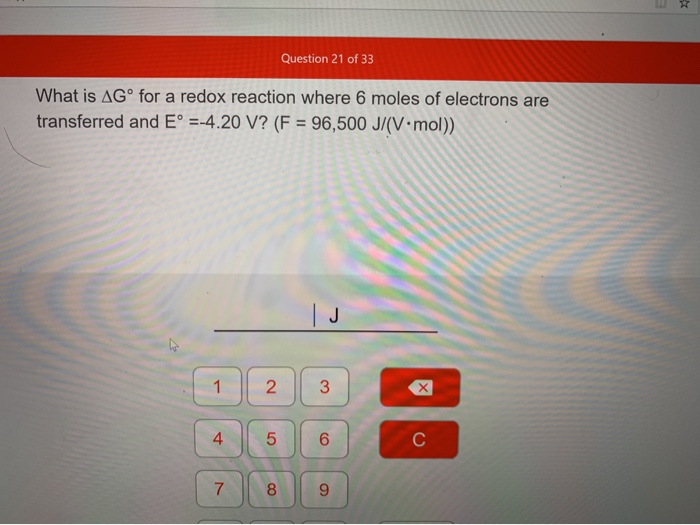Question 21 of 33 What is AGº for a redox reaction where 6 moles of electrons are transferred and E° =-4.20 V? (F = 96,500 J/(V.mol))

We know the formula, ∆G° = - nFE°

Where, ∆G° is the change in free energy at STP

n is number of electron transferred

E° is redox potential of a cell at STP

Given, n=6 mol, F= 96500 J/(V-mol) , E°= -4.20 V

So, ∆G°= -(6 mol) ×( 96500J/(V-mol))× (-4.20V)

= 2,431,800 J (ans)

#### Earn Coin

Coins can be redeemed for fabulous gifts.

Similar Homework Help Questions
• ### Question 22 of 33 What is the equilibrium constant K at 25°C for an electrochemical cell...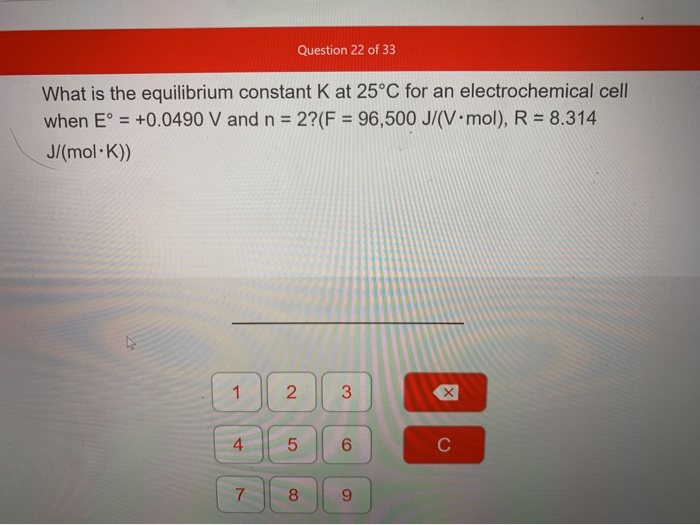Question 22 of 33 What is the equilibrium constant K at 25°C for an electrochemical cell when E° = +0.0490 V and n = 2? (F = 96,500 J/(V.mol), R = 8.314 J/(mol·K))

• ### Free-energy change, AGº, is related to cell potential, Eº, by the equation AG° = -nFE° where...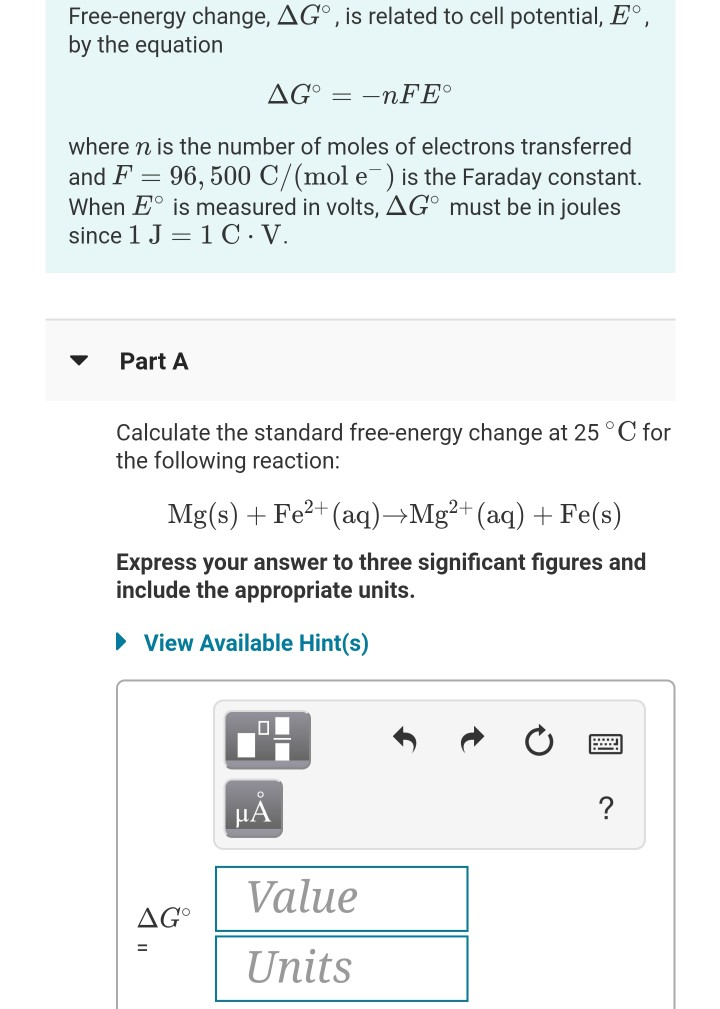Free-energy change, AGº, is related to cell potential, Eº, by the equation AG° = -nFE° where n is the number of moles of electrons transferred and F = 96,500 C/(mol e ) is the Faraday constant. When Eº is measured in volts, AGⓇ must be in joules since 1 J =1C.V. Part A Calculate the standard free-energy change at 25°C for the following reaction: Mg(s) + Fe2+ (aq)Mg2+ (aq) + Fe(s) Express your answer to three significant figures and include...

• ### The equilibrium constant, K. for a redox reaction is related to the standard potential, E, by...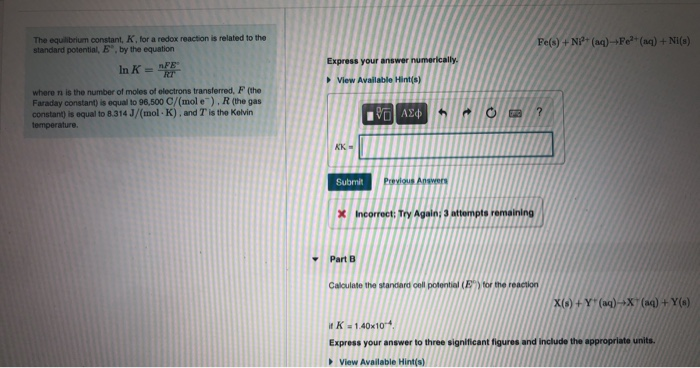The equilibrium constant, K. for a redox reaction is related to the standard potential, E, by the equation Fe(s) + Ni+ (aq) +Fe?+ (aq) + NI(s) FE In K = Express your answer numerically. View Available Hints) where n is the number of moles of electrons transferred, F (the Faraday constant) is equal to 96,500 C/(mole). R (the gas constant) is equal to 8.314 J/(mol-K). and T is the Kelvin temperature. ΟΙ ΑΣΦ h ? KK- Submit Previous Answers *...

• ### For the balanced redox reaction (2 electrons transferred in the balanced reaction): 2Mn2+ (aq) + PbO2...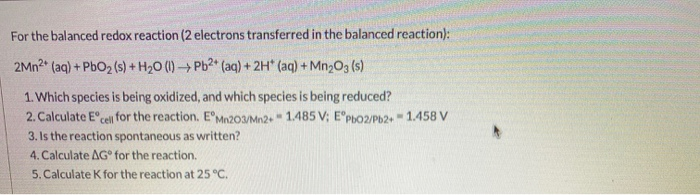For the balanced redox reaction (2 electrons transferred in the balanced reaction): 2Mn2+ (aq) + PbO2 (s) + H20 (1) + Pb2+ (aq) + 2H+ (aq) + Mn203 (s) 1. Which species is being oxidized, and which species is being reduced? 2. Calculate Eºcell for the reaction. EºMn203/Mn2+ - 1.485V; EºPb02/Pb2+ - 1.458 V 3. Is the reaction spontaneous as written? 4. Calculate AGº for the reaction. 5. Calculate for the reaction at 25°C.

• ### The cell potential of a redox reaction occurring in an electrochemical cell under any set of...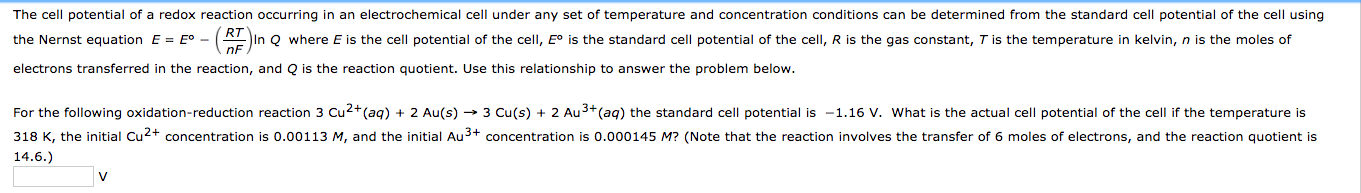The cell potential of a redox reaction occurring in an electrochemical cell under any set of temperature and concentration conditions can be determined from the standard cell potential of the cell using the Nernst equation where E is the cell potential of the cell, E° is the standard cell potential of the cell, R is the gas constant, T is the temperature in kelvin, n is the moles of electrons transferred in the reaction, and Q is the reaction quotient....

• ### What is E°cell (in V) for a redox reaction that has ΔrG° = -308 kJ mol-1...

What is E°cell (in V) for a redox reaction that has ΔrG° = -308 kJ mol-1 at 25°C ? Assume 2 electrons are transferred in the balanced redox reaction.

• ### What is the balanced number of moles of electrons transferred in the following reaction? 3Mn +...

What is the balanced number of moles of electrons transferred in the following reaction? 3Mn + 2AuCl4– → 3Mn2+ + 2Au + 8Cl– Question options: a. 4 b. 2 c. 3 d. 6

• ### The equilibrium constant, K, for a redox reaction is related to the standard potential, Eº, by...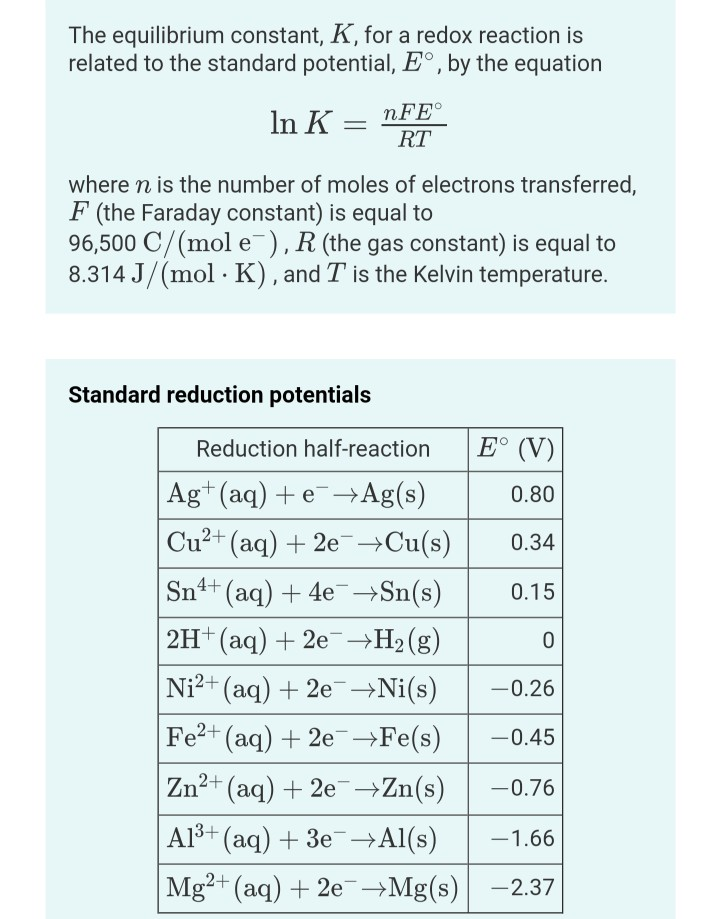The equilibrium constant, K, for a redox reaction is related to the standard potential, Eº, by the equation In K = nFE° RT where n is the number of moles of electrons transferred, F (the Faraday constant) is equal to 96,500 C/(mol e), R (the gas constant) is equal to 8.314 J/(mol · K), and T is the Kelvin temperature. Standard reduction potentials Reduction half-reaction E° (V) Ag+ (aq) + e +Ag(s) 0.80 Cu²+ (aq) + 2e + Cu(s) 0.34...

• ### 6-What is the E°cell (in V) for a redox reaction that has equilibrium constant K =7.8...

6-What is the E°cell (in V) for a redox reaction that has equilibrium constant K =7.8 x 10-2 at 25°C ? Assume 1 electrons are transferred in the balanced redox reaction. Remember: if you want to express an answer in scientific notation, use the letter "E". For example "4.32 x 104" should be entered as "4.32E4". 7--Consider a hypothetical salt of low solubility, "MX", where M+ is a metal cation and X- is a non-metal anion. A voltaic cell was...

• ### QUESTION 4 A balanced redox reaction involves exchange of 2 electrons and generates a potential difference...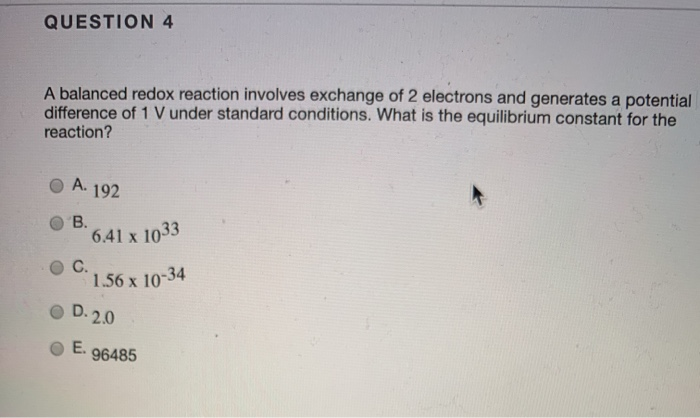QUESTION 4 A balanced redox reaction involves exchange of 2 electrons and generates a potential difference of 1 V under standard conditions. What is the equilibrium constant for the reaction? A. 192 В. 6.41x 1033 С. 1.56 x 10-34 D.2.0 E. 96485